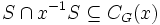# Automorphism sends more than three-fourths of elements to inverses implies abelian

This article is about a result whose hypothesis or conclusion has to do with the fraction of group elements or tuples of group elements satisfying a particular condition.The fraction involved in this case is 3/4
View other such statements

## Statement

Let$G$ be a finite group, and$\sigma$ be an automorphism of$G$, such that the subset:$S := \{ g \mid \sigma(g) = g^{-1} \}$

has size more than three-fourths the size of$G$. Then,$G$ is an abelian group (in particular, a finite abelian group) and$\sigma$ is the inverse map.

## Related facts

### Tightness

There exist examples of non-abelian groups with automorphisms that send exactly 3/4 of the elements to their inverses. For instance:

• In the eight-element quaternion group with elements$i,j,k$ of order four, the map sending$i,j$ to their negatives but sending$k$ to itself, sends exactly 3/4 of the elements to their inverses.
• In the eight-element dihedral group:D8, the identity automorphism sends three-fourths of the elements to their inverses, because$5$ of the non-identity elements have order two.

## Facts used

1. The set of elements that commute with any fixed element of the group, is a subgroup: the so-called centralizer of the element.
2. The set of elements that commute with every element of the group, is a subgroup: the so-called center of the group
3. Subgroup of size more than half is whole group
4. Abelian implies universal power map is endomorphism, in particular the inverse map is an endomorphism. Since the inverse map is also obviously bijective, this also yields that it is an automorphism.
5. The image of a generating set under a homomorphism completely determines the homomorphism.

## Proof

This proof uses a tabular format for presentation. Provide feedback on tabular proof formats in a survey (opens in new window/tab) | Learn more about tabular proof formats|View all pages on facts with proofs in tabular format

### Proof details

Given: A finite group$G$, an automorphism$\sigma$ of$G$.$S$ is the subset of$G$ comprising those$g$ for which$\sigma(g) = g^{-1}$. We are given that$\! |S| > (3/4) |G|$.

To prove:$G$ is abelian and$S = G$, i.e.,$\sigma(g) = g^{-1}$ for all$g \in G$.

Proof: We initially focus on a single element$x \in S$ and try to show that its centralizer is the whole of$G$. We then shift focus to$S$ as a set, show that it is contained in the center, and since the center is a subgroup, it is forced to be all of$G$.

Step no. Assertion/construction Facts used Given data used Previous steps used Explanation Commentary
1 Suppose$x,y \in G$ are such that$x,y,xy$ are all in$S$. Then$xy = yx$ -- Definition of$S$ as the set of elements mapped to their inverses,$\sigma$ is an endomorphism [SHOW MORE] Convert local property of elements going to inverses to local property of pairs commuting.
2 For any$x \in S$, define$x^{-1}S := \{ x^{-1}s : s \in S \}$. We have$S \cap x^{-1}S \subseteq C_G(x)$, where$C_G(x)$ is the centralizer of$x$ in$G$. Step (1) [SHOW MORE] Toward establishing a large centralizer for fixed but arbitrary element of$S$ -- use local property of elements going to inverses.
3 For any$x \in S$, the intersection$S \cap x^{-1}S$ has size greater than$|G|/2$$|S| > (3/4)|G|$ [SHOW MORE] Toward establishing a large centralizer for fixed but arbitrary element of$S$ -- use size constraints and combinatorial formula.
4 For any$x \in S$, the subgroup$C_G(x) = \{ y \in G \mid xy = yx \}$ is equal to the whole group$G$ Facts (1), (3) Steps (2), (3) [SHOW MORE] Complete establishing that a fixed but arbitrary element of$S$ has centralizer the whole group -- use that big enough subgroups must contain everything.
5$S$ is contained in the center$Z(G)$ of$G$ Step (4) [SHOW MORE] Shift focus to$S$ as a set.
6$G$ equals its own center, hence is abelian Facts (2), (3)$|S| > (3/4)|G|$ Step (5) [SHOW MORE] Clinch -- use that big enough subgroups must contain everything.
7$S$ is a generating set for$G$. Fact (3)$|S| > (3/4)|G|$ -- [SHOW MORE]
8$S = G$, i.e.,$\sigma(g) = g^{-1}$ for all$g \in G$. Facts (4), (5) -- Steps (6), (7) [SHOW MORE]

Steps (6) and (8) clinch the proof.